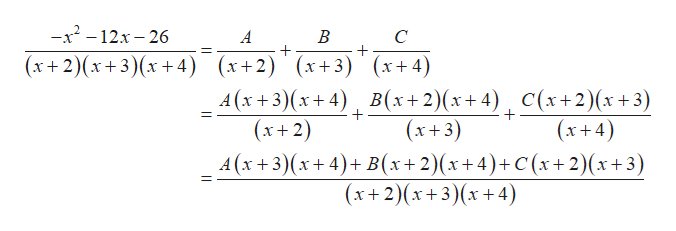# Given the partial decomposition of the rational function f(x), find A, B and C. f(x)=(-x^(2)-12x-26)/((x+2)(x+3)(x+4))=(A)/(x+2)+(B)/(x+3)+(C)/(x+4)

Question
1 views

Given the partial decomposition of the rational function f(x), find A, B and C.

f(x)=(-x^(2)-12x-26)/((x+2)(x+3)(x+4))=(A)/(x+2)+(B)/(x+3)+(C)/(x+4)

check_circle

Step 1

The constant value in partial decomposition of the ratio...help_outlineImage Transcriptionclose-x2-12x-26 B C (x+2)(x+3)(x+4) (x+2) (x+3) x4) A (x+3 x4) B(x2)(x+ 4) (x2) C(x+2)(x3) + + (x+3) (x+4) A(x+3) (x+4)+ B(x2)(x+4)+C (x+2)(x+3) (x+2)(x+3)(x4) fullscreen

### Want to see the full answer?

See Solution

#### Want to see this answer and more?

Solutions are written by subject experts who are available 24/7. Questions are typically answered within 1 hour.*

See Solution
*Response times may vary by subject and question.
Tagged in

### Other